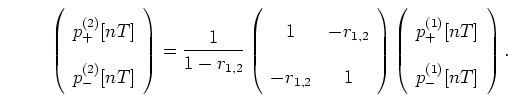Back to Kemp Acoustics HomeNext: Multimodal scattering matrix Up: Multimodal reflections Previous: Multimodal input impulse response   Contents

# Issues in multimodal bore reconstruction

Now we will discuss possible approaches to the inclusion of multimodal calculations in acoustic pulse reflectometry bore reconstruction. This procedure has yet to be implemented because of the difficulties arising from the frequency dependent reflections and the additional computation power required for computing multimodal behaviour in an already computationally expensive procedure. This section therefore is a review of the theoretical background on which future work may be based, and a discussion of the problems and possible solutions. To start, it is useful to present a brief summary of the plane wave approximation algorithm set out in chapter 5.

In an acoustic pulse reflectometry measurement the first step is the experimental measurement of the input impulse response. If a forward going ideal impulse enters the input, by definition the reflections are the input impulse response sequence. We therefore know both the forward and backward going waves at the input. The plane reflection coefficient of equation (2.20) gives the amplitude of the primary reflection of an incident wave in terms of the change in cross-section. The plane reflection coefficient (and therefore the change in cross-section) are found from the the ratio of the first entries in the forward and backward going pressure vectors respectively, since they correspond to a primary reflection.

Scattering equations are then used to find the forward and backward going waves in the next cylindrical section. This process is then repeated, working out the forward and backward going waves and the change in bore at each step along the bore in turn untill the output end is reached. The scattering equations used are equations (5.11), (5.17) and (5.18):(6.17)(6.18)(6.19)

Here surfaces 1 and 2 are on the left and right of the initial discontinuity respectively and section 3 is a distanceto the right of surface 2 (see figure 5.1).

For a multimodal theory, the reflection coefficient is frequency dependent, meaning that the primary reflection of an impulse from the first step is not impulsive, but is spread out over a finite time. Ideally, the primary reflections then should not simply be calculated from the ratio of the first time step in the forward and backward going pressure vectors. A rigorous analysis should look at the plane reflectance, the frequency spectrum of the impulse response of the step in the absence of secondary reflections. As with figure 6.4 the plane wave approximation reflection coefficient corresponding to the cross-section change would then be equal to the low frequency limiting behaviour of this plane reflectance. The problem with this is that the secondary reflections are present in the input impulse response and are as yet unknown; after the first time step the primary reflection from the first cross-section change is swamped by information about the rest of the instrument.

Further investigation is necessary to see if the plane wave approximation reflection coefficient from the first time step of the primary reflection can give accurate enough results. The bore reconstruction algorithm may still incorporate multimodal effects by the use of a multimodal scattering equation. While the first change in cross-section would be calculated without multimodal effects, the forward and backward going waves on the other side of the step would be calculated including the loss of energy to higher modes. The value of the primary reflection from the next step would therefore be effected by the multimodal treatment.

Subsections
Back to Kemp Acoustics HomeNext: Multimodal scattering matrix Up: Multimodal reflections Previous: Multimodal input impulse response   Contents
Jonathan Kemp 2003-03-24